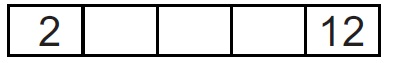# Think more, mathematicians

Algebra Level 2In a list of five numbers, the first number is 2 and the last number is 12. The product of the first three numbers is 30, the product of the three in the middle is 90 and the product of the last three numbers is 360. Which number is in the centre of the list?

×